# Check if Number is not Greater than 0 in JavaScriptLast updated: Jan 6, 2023
3 min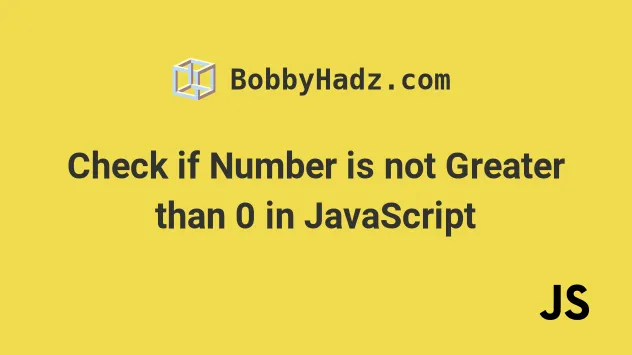## #Check if a Number is not Greater than 0 in JavaScript

Use the less than or equals to `<=` operator to check if a number is not greater than zero, e.g. `if (num <= 0) {}`.

The comparison will return `true` if the number is not greater than zero and `false` otherwise.

index.js
```Copied!```const num = -5;

if (num <= 0) {
// 👇️ this runs
console.log('✅ number is not greater than 0');
} else {
console.log('⛔️ number is greater than 0');
}
``````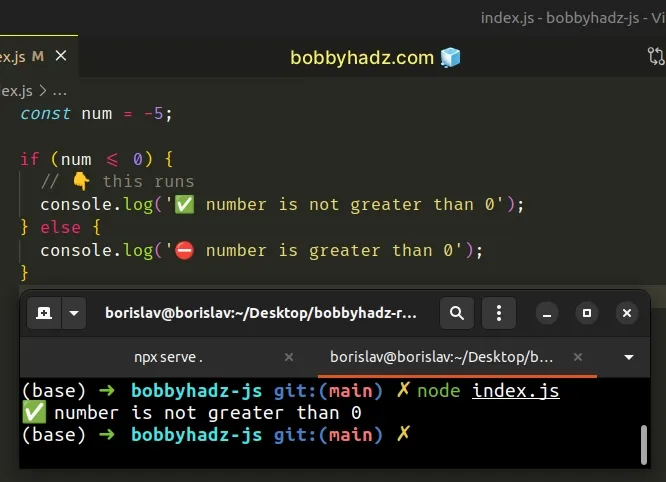The less than or equals to `<=` operator returns `true` if the value to the left is less than or equal to the value to the right.

If the number is less than or equal to `0`, then it's not greater than `0`.

If you have to check if a number is not greater than `0` often, define a reusable function.

index.js
```Copied!```function isNotGreaterThanZero(num) {
return num <= 0;
}

console.log(isNotGreaterThanZero(0)); // 👉️ true
console.log(isNotGreaterThanZero(5)); // 👉️ false
console.log(isNotGreaterThanZero(-10)); // 👉️ true

if (isNotGreaterThanZero(-5)) {
// 👇 this runs
console.log('The number is not greater than zero');
} else {
console.log('The number is greater than zero');
}
``````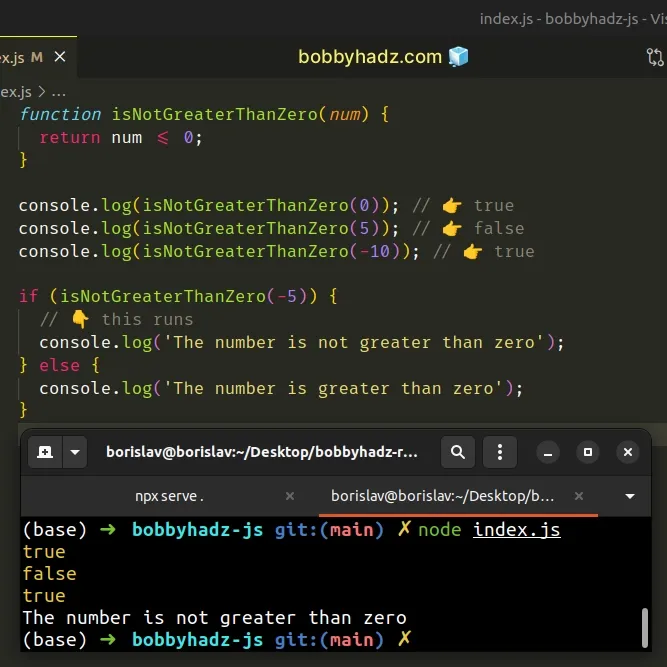The `isNotGreaterThanZero` function takes a number as a parameter and returns `true` if the number is not greater than zero and `false` otherwise.

## #Check if a Number is not Greater than 0 using logical NOT (!) operator

Alternatively, you can use the logical NOT (!) operator.

If the number is not greater than `0`, the expression returns `true`, otherwise `false` is returned.

index.js
```Copied!```const num = -5;

if (!(num > 0)) {
// 👇️ this runs
console.log('✅ number is not greater than 0');
} else {
console.log('⛔️ number is greater than 0');
}
``````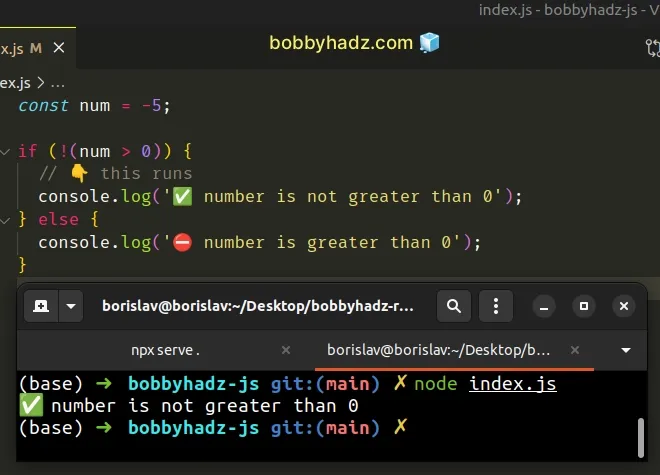We used the logical NOT (!) operator to negate the greater than sign.

Note that we had to wrap the condition in parentheses.

Had we not done that, we would have used the logical NOT (!) operator to convert the `num` variable to a boolean and negate the result.
index.js
```Copied!```const num = -5;

// ⛔️ BAD (don't do this)
console.log(!num > 0); // 👉️ false
``````

The code sample above doesn't use parentheses, so we convert the `num` variable to a boolean and negate it.

index.js
```Copied!```const num = -5;

console.log(!num); // 👉️ false
``````

All numbers other than `0` are truthy values, so when converted to a boolean, they get converted to `true` and when negated, they become `false`.

Instead, we want to wrap the expression in parentheses.

index.js
```Copied!```const num = -5;

if (!(num > 0)) {
console.log('✅ number is not greater than 0');
} else {
console.log('⛔️ number is greater than 0');
}
``````

Now we first check if the number is greater than `0`, then convert the result to a boolean and negate it.

If the number is greater than `0`, the expression returns `true` and when the logical NOT (!) operator is applied, `false` is returned, so the `else` block runs.

The `if` block runs only if the number is not greater than `0`.

If you have to do this often, define a reusable function.

index.js
```Copied!```const num = -5;

function isNotGreaterThanZero(num) {
return !(num > 0);
}

console.log(isNotGreaterThanZero(0)); // 👉️ true
console.log(isNotGreaterThanZero(5)); // 👉️ false
console.log(isNotGreaterThanZero(-10)); // 👉️ true

if (isNotGreaterThanZero(-5)) {
// 👇 this runs
console.log('The number is not greater than zero');
} else {
console.log('The number is greater than zero');
}
``````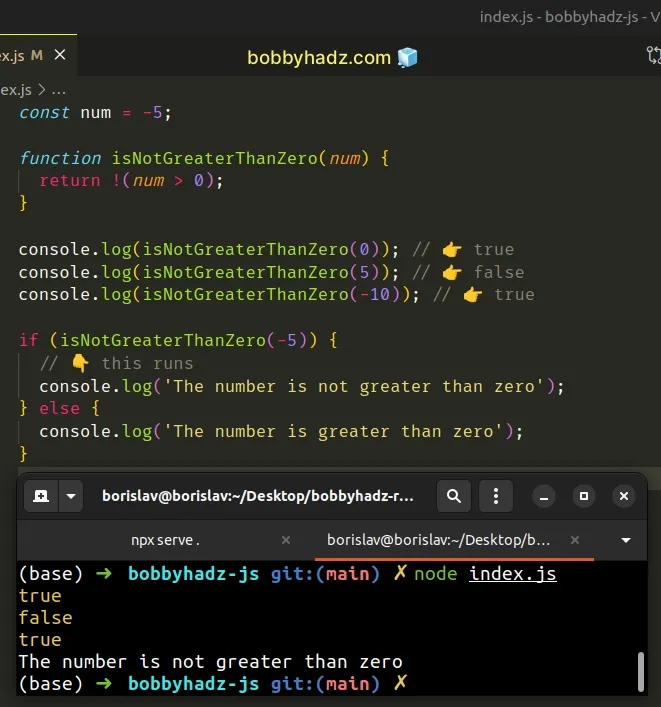The function takes a number as a parameter and returns `true` if the number is not greater than `0` and `false` otherwise.

Which approach you pick is a matter of personal preference. I'd use the `if (num <= 0) {}` comparison as I find it more readable and intuitive.